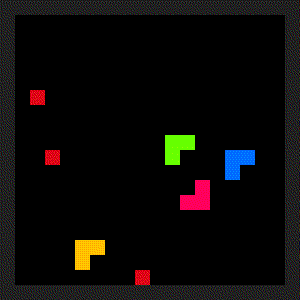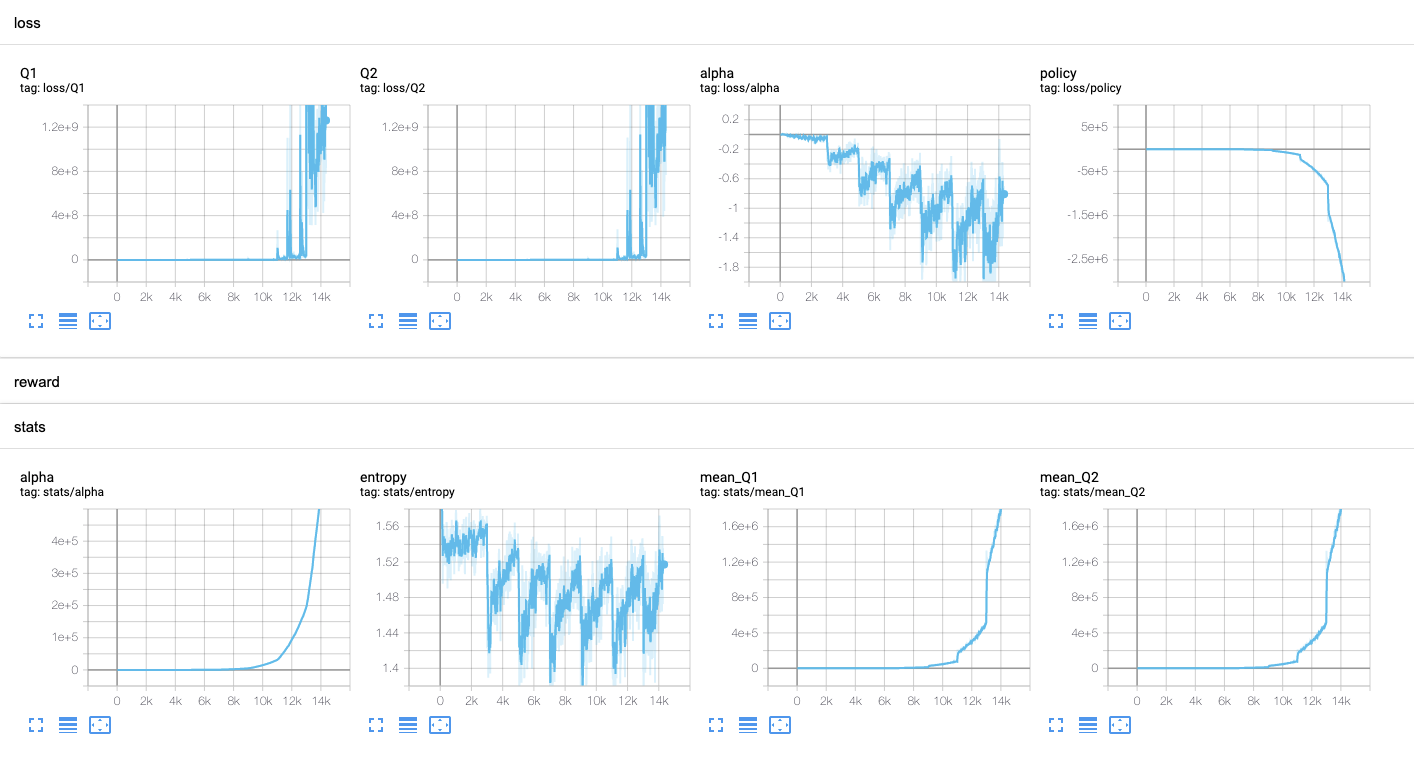# Diary - How to solve discrete-SAC loss explosion problem?

One of the most strong advantage of Soft-Actor-Critic (SAC) reinforcement learning method is the robustness about hyperparameters. Almost every cases, this algorithm shows outstanding performance regardless of environment type. Indeed, SAC was proposed for handling continuous action space. We have to modify original SAC for applying in discrete action space environment like Atari games. Followng link shows the detail of discrete SAC.

Discrete SAC

I applied this method to solve Snake game. I expected that SAC easily solve this task.However, the critic loss started to exploding.To solve this problem, we need to tune the entropy target ratio. Then what is the entropy target ratio?

Contrast to the original SAC (for continous action space), Discrete SAC can calculate entropy easily because we exactly know the probability of each action. Therefore, the discrete SAC set the target entropy as following

$\tilde{H}=-\sum_{N(A)}\frac{1}{N(A)}log\frac{1}{N(A)}=-log\frac{1}{N(A)}$

It is the maximum entropy when the action distribution is same as the uniform distribution. It is really strict condition that be never obtained. So the entropy target ratio is multiplied to the uniform target entropy. In the discrete SAC paper, it was set as 0.98.

However, this value must be set as lower value in the environment like the snake game. The snake game has 5 actions; none, left, right, up, down. But only three action can be affect to the snake. So 0.98 is still strict for the snake game, and it’s the main reason of the explosion of critic loss. We found that lower than 0.8 is proper for the snake game.

Even if you set much lower value, it should be work. But keep it in mind that it makes losing the main advantage of SAC which is the powerful exploration.